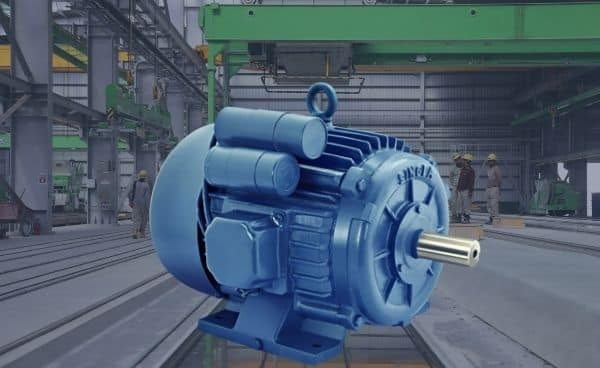# Why Single Phase Induction Motor is not Self Starting? & How to make it self starting.According to double field revolving theory, we can resolve any alternating quantity into two components. Each component has a magnitude equal to the half of the maximum magnitude of the alternating quantity, and both these components rotate in the opposite direction to each other. For example – a flux, φ can be resolved into two components.

Each of these components rotates in the opposite direction i. e if one φm/2 is rotating in a clockwise direction then the other φm / 2 rotates in an anticlockwise direction.

When we apply a single-phase AC supply to the stator winding of single phase induction motor, it produces its flux of magnitude, φm. According to the double field revolving theory, this alternating flux, φm is divided into two components of magnitude φm/2. Each of these components will rotate in the opposite direction, with the synchronous speed, Ns.
Let us call these two components of flux as forwarding component of flux, φf and the backward component of flux, φb. The resultant of these two components of flux at any instant of time gives the value of instantaneous stator flux at that particular instant.

Now at starting condition, both the forward and backward components of flux are exactly opposite to each other. Also, both of these components of flux are equal in magnitude. So, they cancel each other, and hence the net torque experienced by the rotor at the starting condition is zero. So, the single-phase induction motors are not self-starting motors.

Methods for Making Single Phase Induction as Self Starting Motor

From the above topic, we can easily conclude that the single-phase induction motors are not self-starting because the produced stator flux is alternating in nature and at the starting, the two components of this flux cancel each other and hence there is no net torque. The solution to this problem is that if we make the stator flux rotating type, rather than alternating type, which rotates in one particular direction only. Then the induction motor will become self-starting.

Now for producing this rotating magnetic field, we require two alternating flux, having some phase difference angle between them. When these two fluxes interact with each other, they will produce a resultant flux. This resultant flux is rotating in nature and rotates in space in one particular direction only.
Once the motor starts running, we can remove the additional flux. The motor will continue to run under the influence of the main flux only. Depending upon the methods for making asynchronous motor as Self Starting Motor, there are mainly four types of single-phase induction motor namely,

1. Split phase induction motor,
2. Capacitor start inductor motor,
3. Capacitor start capacitor run induction motor,